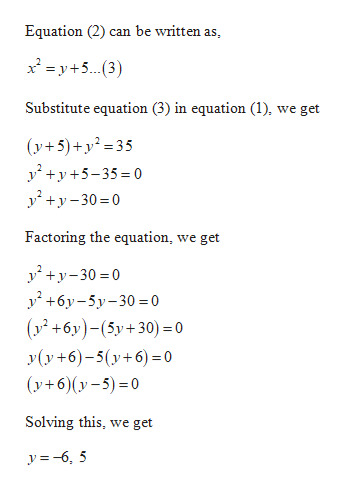# Solve the following system of equations:y235y=x2-5

Question
3 views
check_circle

Step 1

Given:

Step 2

Calculation:

We can solve the given system of equatio...help_outlineImage TranscriptioncloseEquation (2) can be written as, 2y+(3) Substitute equation (3) in equation (1), we get (y+5)+y 35 Factoring the equation, we get 00 6 0= 0 (y2+6y)-(5y+30) = 0 y(y+6)-5(y6= 0 (y+6)(y-5)0 Solving this, we get y-6, 5 fullscreen

### Want to see the full answer?

See Solution

#### Want to see this answer and more?

Solutions are written by subject experts who are available 24/7. Questions are typically answered within 1 hour.*

See Solution
*Response times may vary by subject and question.
Tagged in

### Algebra